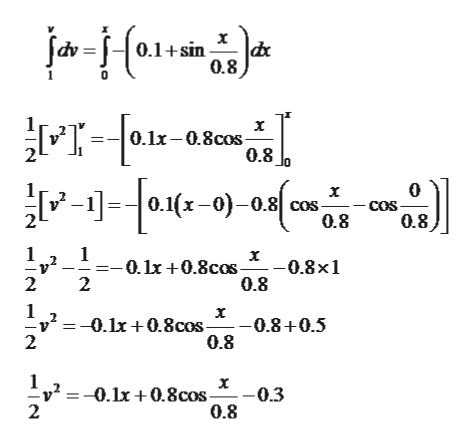# Based on experimental observations, the acceleration of a particle is defined by the relation a = –(0.1 + sin x/b), where a and x are expressed in m/s2 and meters, respectively. Know that b = 0.80 m and that v = 1 m/s when x = 0. Determine the velocity of the particle when x = –1 m.

Question

Based on experimental observations, the acceleration of a particle is defined by the relation a = –(0.1 + sin x/b), where a and x are expressed in m/s2 and meters, respectively. Know that b = 0.80 m and that v = 1 m/s when x = 0.

Determine the velocity of the particle when x = –1 m.

check_circle

Step 1

The acceleration of the particle is given by the equation:

Where (a) in m/s2 and (x) is in meters.

Also the given condition is that: v =1m/s when x= 0 and b = 0.8 m.

Step 2

The basic kinematics relation is given by the equation, if a = a(x)  then,

Step 3

Now, integrate the equation within t...help_outlineImage Transcriptionclose0.1+sin |dx '0.8 0.1x-0.8cos 0.8 x [ -1]=- lx-0)-08| соз. COs 0.8 0.8 1 1 --0.lx +0.8cos- 2 0.8x1 0.8 2 х -0.8+0.5 0.8 v20.10.8cos- 2 х 0.3 0.8 - -0.1x +0.8cos 2 fullscreen

### Want to see the full answer?

See Solution

#### Want to see this answer and more?

Solutions are written by subject experts who are available 24/7. Questions are typically answered within 1 hour.*

See Solution
*Response times may vary by subject and question.
Tagged in

### Mechanical Engineering